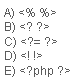### PHP Basics Multiple Choice Questions

1)
What PHP stands for?

A) Hypertext Preprocessor
B) Pre Hypertext Processor
C) Pre Hyper Processor
D) Pre Hypertext Process

2)
Which of the following tags is not a valid way to begin and end a PHP code block?3)
How does the identity operator === compare two values?

A) It converts them to a common compatible data type and then compares the resulting values
B) It returns True only if they are both of the same type and value
C) If the two values are strings, it performs a lexical comparison
D) It bases its comparison on the C strcmp function exclusively
E) It converts both values to strings and compares them

4)
Under what circumstance is it impossible to assign a default value to a parameter while declaring a function?

A) When the parameter is Boolean
B) When the function is being declared as a member of a class
C) When the parameter is being declared as passed by reference
D) When the function contains only one parameter
E) Never

5)

A) Pond-sign
B) Yen-sign
C) Dollar-sign
E) Euro-sign

6)
What is the value displayed when the following is executed? Assume that the code was executed using the following URL:

testscript.php?c=25

<?php
function process(\$c, \$d = 25)
{
global \$e;
\$retval = \$c + \$d - \$_GET['c'] - \$e;
return \$retval;
}
\$e = 10;
echo process(5);
?>

A) 25
B) -5
C) 10
D) 5
E) 0

7)
PHP is an open source software

A) True
B) False

8)
Which of the following is not valid PHP code?

A) \$_10
B) \${“MyVar”}
C) &\$something
D) \$10_somethings
E) \$aVaR

9)
What is the difference between print() and echo()?

A) print() can be used as part of an expression, while echo() can’t
B) echo() can be used as part of an expression, while print() can’t
C) echo() can be used in the CLI version of PHP, while print() can’t
D) print() can be used in the CLI version of PHP, while echo() can’t
E) There’s no difference: both functions print out some text!

10)
PHP runs on different platforms (Windows, Linux, Unix, etc.)

A) True
B) False